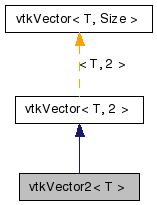vtkVector2< T > Class Template Reference

#include <vtkVector.h>

Inheritance diagram for vtkVector2< T >:[legend]
Collaboration diagram for vtkVector2< T >:[legend]

Detailed Description

template<typename T> class vtkVector2< T >

Definition at line 97 of file vtkVector.h.

Public Member Functions

vtkVector2 (const T &x=0, const T &y=0)
vtkVector2 (const T *init)
void SetX (const T &x)
void SetY (const T &y)
void Set (const T &x, const T &y)
const T & GetX () const
const T & X () const
const T & GetY () const
const T & Y () const

Constructor & Destructor Documentation

template<typename T>
 vtkVector2< T >::vtkVector2 ( const T & x = 0, const T & y = 0 ) [inline]

Definition at line 100 of file vtkVector.h.

template<typename T>
 vtkVector2< T >::vtkVector2 ( const T * init ) [inline, explicit]

Definition at line 106 of file vtkVector.h.

Member Function Documentation

template<typename T>
 void vtkVector2< T >::Set ( const T & x, const T & y ) [inline]

Set the x and y components of the vector.

Definition at line 112 of file vtkVector.h.

template<typename T>
 void vtkVector2< T >::SetX ( const T & x ) [inline]

Set the x component of the vector, i.e. element 0.

Definition at line 120 of file vtkVector.h.

template<typename T>
 const T& vtkVector2< T >::GetX ( ) const [inline]

Get the x component of the vector, i.e. element 0.

Definition at line 124 of file vtkVector.h.

template<typename T>
 const T& vtkVector2< T >::X ( ) const [inline]

Get the x component of the vector, i.e. element 0.

Definition at line 125 of file vtkVector.h.

template<typename T>
 void vtkVector2< T >::SetY ( const T & y ) [inline]

Set the y component of the vector, i.e. element 1.

Definition at line 129 of file vtkVector.h.

template<typename T>
 const T& vtkVector2< T >::GetY ( ) const [inline]

Get the y component of the vector, i.e. element 1.

Definition at line 133 of file vtkVector.h.

template<typename T>
 const T& vtkVector2< T >::Y ( ) const [inline]

Get the y component of the vector, i.e. element 1.

Definition at line 134 of file vtkVector.h.

The documentation for this class was generated from the following file:

Generated on Wed Aug 24 12:17:10 2011 for VTK by1.5.6